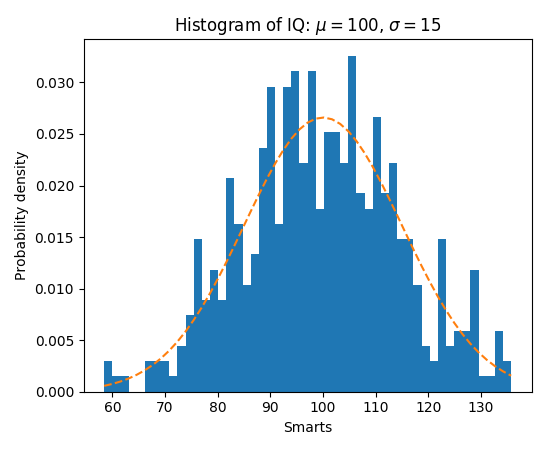Travis-CI:statistics example code: histogram_demo_features.py¶"""
=========================================================
Demo of the histogram (hist) function with a few features
=========================================================

In addition to the basic histogram, this demo shows a few optional
features:

* Setting the number of data bins
* The normed flag, which normalizes bin heights so that the
integral of the histogram is 1. The resulting histogram is an
approximation of the probability density function.
* Setting the face color of the bars
* Setting the opacity (alpha value).

Selecting different bin counts and sizes can significantly affect the
shape of a histogram. The Astropy docs have a great section on how to
select these parameters:
http://docs.astropy.org/en/stable/visualization/histogram.html
"""

import numpy as np
import matplotlib.mlab as mlab
import matplotlib.pyplot as plt

np.random.seed(0)

# example data
mu = 100  # mean of distribution
sigma = 15  # standard deviation of distribution
x = mu + sigma * np.random.randn(437)

num_bins = 50

fig, ax = plt.subplots()

# the histogram of the data
n, bins, patches = ax.hist(x, num_bins, normed=1)

# add a 'best fit' line
y = mlab.normpdf(bins, mu, sigma)
ax.plot(bins, y, '--')
ax.set_xlabel('Smarts')
ax.set_ylabel('Probability density')
ax.set_title(r'Histogram of IQ: $\mu=100$, $\sigma=15$')

# Tweak spacing to prevent clipping of ylabel
fig.tight_layout()
plt.show()

Keywords: python, matplotlib, pylab, example, codex (see Search examples)